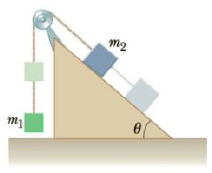Chapter 4, Problem 70P

Chapter
Section
Textbook Problem

Objects of masses m1 = 4.00 kg and m2 = 9.00 kg are connected by a light string dial passes over a frictionless pulley as in Figure P4.70. The object m1 is held at rest on the floor, and m2 rests on a fixed incline of θ = 40.0°. The objects are released from rest, and m2 slides 1.00 m down the incline in 4.00 s. Determine (a) the acceleration of each object, (b) the tension in the string, and (c) the coefficient of kinetic friction between m2 and the incline.Figure P4.70

(a)

To determine
The acceleration.

Explanation

Given info: The masses are m1 = 4.00 kg and m2 = 9.00 kg. Angle of inclination is 40ο . The mass m2 slides 1.00 m in 4 s.

From Newton’s equation of motion, the distance is,

s=ut+12at2

• u is the initial velocity.
• t is the time taken.
• a is the acceleration.

Substitute 0 m/s for u to get s.

s=12at2

On Re-arranging, the acceleration is,

a=2st2

Substitute 1

(b)

To determine
The tension in the string.

(c)

To determine
The co-efficient of kinetic friction.

Still sussing out bartleby?

Check out a sample textbook solution.

See a sample solution

The Solution to Your Study Problems

Bartleby provides explanations to thousands of textbook problems written by our experts, many with advanced degrees!

Get Started

Find more solutions based on key concepts